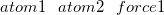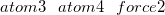Q-Chem 4.3 User’s Manual

# 9.11 The EFEI Method

The EFEI (External Force is Explicitly Included) method was introduced by Marx and co-workers ([451, 452]) and allows to impose mechanical forces on pairs of atoms. An EFEI calculation is a geometry optimization in which a constant that is equal to the external force is added to the nuclear gradient of two atoms specified by the user. The external force is applied along the vector connecting the two atoms, thus driving them apart. The geometry optimization converges when the restoring force of the molecule is equal to the external force. The EFEI method can also be used in AIMD simulations, in which case the force is added in every time step.

Hence, the EFEI method can be applied in combination with geometry optimizations or AIMD simulations. The basis syntax for specifying EFEI calculations is the following:

\$efei\$end

atom1 and atom2 are the indices of the atoms on which a force is applied. force1 is the force in nanonewton (nN). If this value is positive, a mechanical force of magnitude force1 drives the atoms apart. If it is negative, an attractive force acts between the atoms. Optionally, more pairs of atoms that are subject to a force can be specified by adding lines in the \$efei section.

Example 9.208  EFEI calculation of hydrogen peroxide with a constant force of 5 nN driving the oxygen atoms apart

```\$molecule
0 1
O        -0.7059250062   -0.1776745132   -0.0698000882
O         0.7059250062    0.1776745132   -0.0698000882
H         1.0662092915   -0.5838921799    0.4181150580
H        -1.0662092915    0.5838921799    0.4181150580
\$end

\$rem
jobtype         opt
exchange        b3lyp
basis           6-31G*
\$end

\$efei
1 2 5
\$end
```